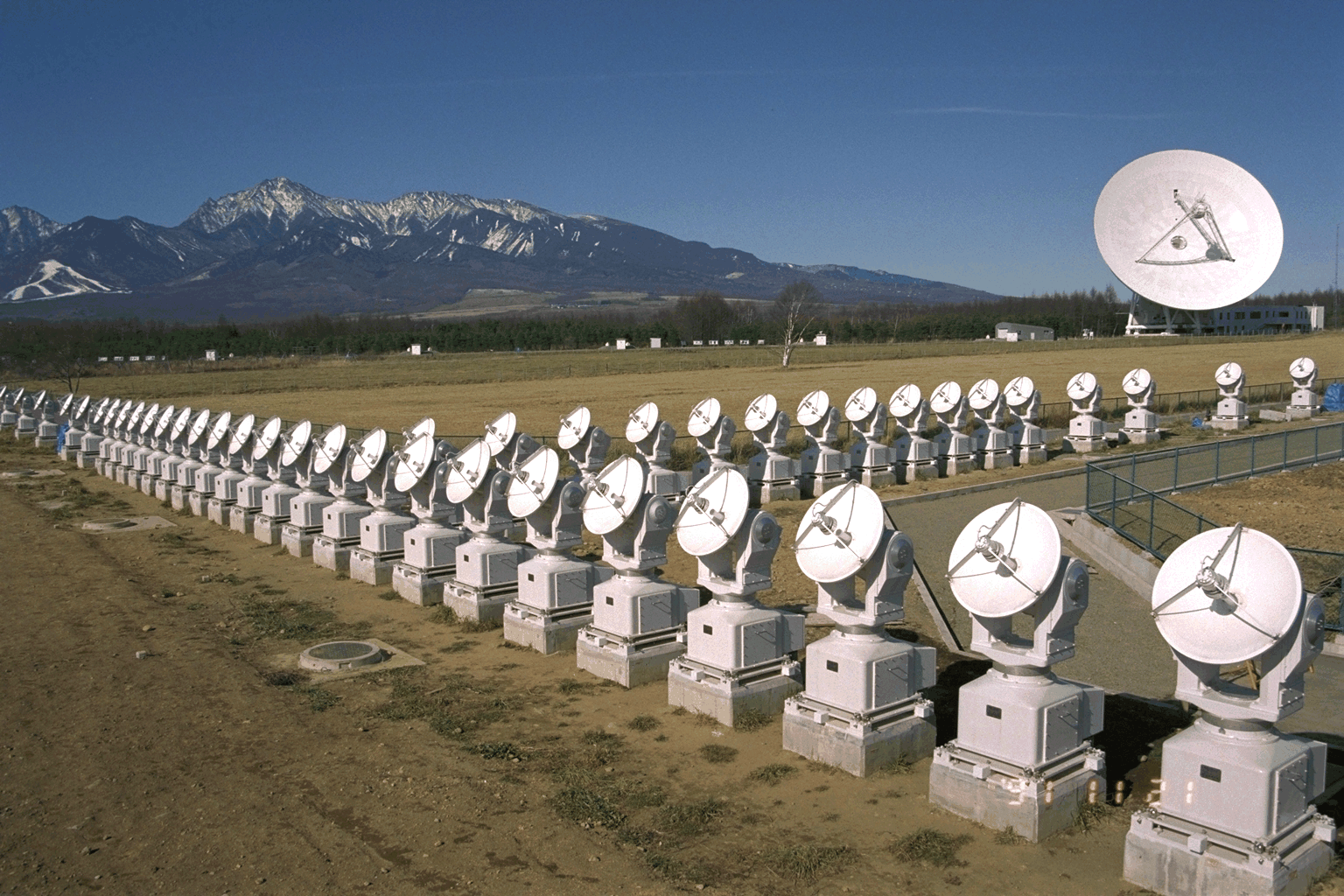# \$BLnJU;3EEGH%X%j%*%0%i%U(B

\$BLnJU;3EEGH%X%j%*%0%i%U!J(BNoRH\$B!K\$O!"(B \$BB@M[4QB,@lMQ\$NEEGHK>1s6@(B \$B\$G\$9!#%X(B \$B%j%*\$OB@M[\$r!"%0%i%U\$O;#A|AuCV\$r0UL#\$7\$^\$9!#K>1s6@\$H\$O\$\$\$C\$F(B \$B\$b7B(B80cm\$B\$N%Q%i%\%i%"%s%F%J(B84\$B4p\$,El@>(B490m\$B!"Fn(B \$BKL(B220m\$B\$N(BT\$B;z7?\$N@~>e\$KG[CV\$5\$l\$?\$b\$N\$G\$9!#(B1990\$BG/EY\$+\$i(B2 \$BG/4V\$G!"Am9)Hq(B18\$B2/1_\$r\$+\$1\$F7z@_\$7!"(B1992\$BG/(B4\$B7n\$K:G=i\$NB@M[(B \$BEEGH2hA|\$N;#1F\$K@.8y\$7!"F1G/(B6\$B7nKv\$h\$jKhF|(B8\$B;~4V\$NO"B34QB,\$r(B \$B9T\$C\$F\$\$\$^\$9!#(B

 \$B4QB,<~GH?t(B 17GHz\$B!J:81&N>1_JPGH!K(B34GHz\$B!J6/EY\$N\$_!K(B \$B4QB,;kLn(B \$BB@M[A4LL(B \$B6u4VJ,2rG=(B 10\$BIC3Q!J(B17GHz\$B!K(B5\$BIC3Q!J(B34GHz\$B!K(B \$B;~4VJ,2rG=(B 0.1\$BIC!J3hF0;~!K(B1\$BIC!J@E2:;~!K(B

\$BLnJU;3EEGH%X%j%*%0%i%U\$O43>D7W\$J\$N\$G!"
\$B!!B@M[\$O2f!9\$N@83h\$N%(%M%k%.!<8;\$G\$"\$j!"0lJ}!"E7J83X\$K\$H\$C\$F(B \$B\$O\$=\$NI=LL\$N3hF0\$,>\:Y\$K4QB,\$G\$-\$kM#0l\$N91@1\$G\$9!#3hF0;~4V\$O(B1 \$BIC0J2<\$N\$b\$N\$+\$i(B10\$BG/0J>e\$K\$o\$?\$k\$b\$N\$^\$G\$5\$^\$6\$^\$G\$9!#EEGH(B \$B%X%j%*%0%i%U\$OB@M[I=LL\$NEEGH\$NL@\$k\$5\$r]\$N4QB,\$r9T\$C\$F\$\$\$^\$9!#(B \$B!!B@M[3hF0\$O(B11\$BG/\$N<~4|\$GBg\$-\$/JQF0\$7!"@>Nq(B2000\$BG/\$K\$=\$N6KBg(B \$B\$KC#\$7\$^\$9!#9uE@\$N?t\$,A}\$(!"\$=\$N<~\$j\$GH/@8\$9\$kGzH/8=>]!JB@M[(B \$B%U%l%"!K\$NIQEY\$b6KBg\$K\$J\$j\$^\$9!#GzH/\$N:]\$K%(%M%k%.!<\$N9b\$\$N3(B \$B;R\$,:n\$i\$l!"\$=\$l\$,9uE@<'>l\$K4,\$-IU\$\$\$FHs>o\$K6/\$\$EEGH\$rH/@8\$7(B \$B\$^\$9!#\$3\$NEEGH\$rB*\$(\$F!"9b%(%M%k%.!e\$2\$i\$l!"\$5\$i\$K4{\$K(B8\$BG/0J>eB@M[\$N4QB,(B \$B\$r9T\$C\$F\$\$\$k1'Ch2J3X8&5f=j\$N!V\$h\$&\$3\$&!W1R@1\$H\$H\$b\$K!"B@M[%U(B \$B%l%"\$NFf2r\$-\$,\$G\$-\$k\$b\$N\$H4|BT\$7\$F\$\$\$^\$9!#(B

\$B;29MJ88%(B
\$B2V2,MG0lO:(B \$B!VB@M[%U%l%"\$rEEGH\$G2rL@\$9\$k!W(B, \$BE7J87nJs(B, 88\$B4,(B5\$B9f(B, 193-198, (1995)
Nakajima et al. "The Nobeyama Radioheliograph", Proc. of the IEEE, 82, 705, (1994)
Takano et al., "An Upgrade of Nobeyama Radioheliograph to a Dual-Frequency (17 and 34 GHz) System" in "Coronal Physics from Radio and Space Observations, Lect. Notes Phys.", (Belrin: Springer-Verlag) 183 (1997)
"The Nobeyama Radioheliograph -- A Collection of Papers on Initial Results and Instrumentation", NRO Report 357 (1994)
"New Look at the Sun with Emphasis on Advanced Observations of Coronal Dynamics and Flares", Proc. of Kofu Symp. NRO Report 360 (1994)

 \$BA0(B \$B%.%c%i%j!<%[!<%`(B \$B